# Maharashtra Board 10th Class Maths Part 1 Practice Set 6.1 Solutions Chapter 6 Statistics

Balbharti Maharashtra State Board Class 10 Maths Solutions covers the Practice Set 6.1 Algebra 10th Class Maths Part 1 Answers Solutions Chapter 6 Statistics.

## Practice Set 6.1 Algebra 10th Std Maths Part 1 Answers Chapter 6 Statistics

Question 1.
The following table shows the number of students and the time they utilized daily for their studies. Find the mean time spent by students for their studies by direct method.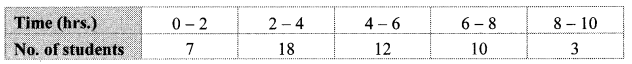Solution: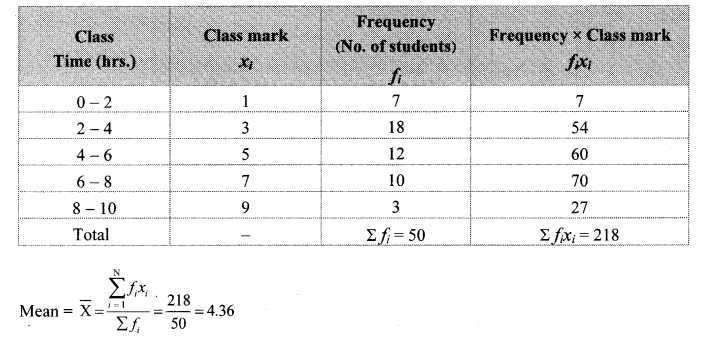∴ The mean of the time spent by the students for their studies is 4.36 hours.

Question 2.
In the following table, the toll paid by drivers and the number of vehicles is shown. Find the mean of the toll by ‘assumed mean’ method.Solution:
Let us take the assumed mean (A) = 550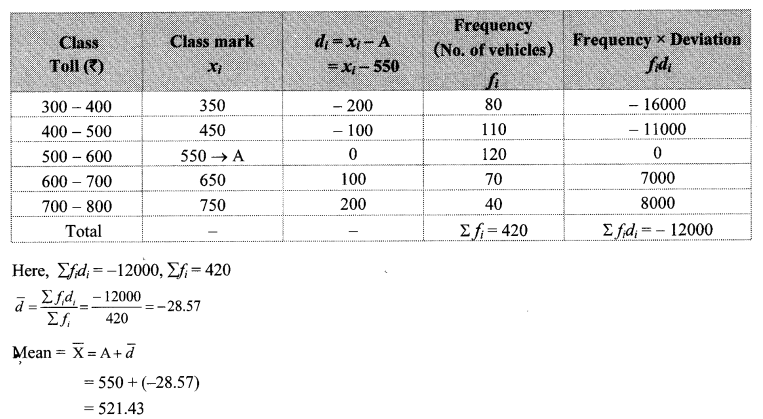∴ The mean of the toll paid by the drivers is ₹ 521.43.

Question 3.
A milk centre sold milk to 50 customers. The table below gives the number of customers and the milk they purchased. Find the mean of the milk sold by direct method.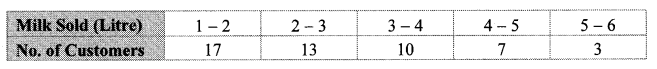Solution: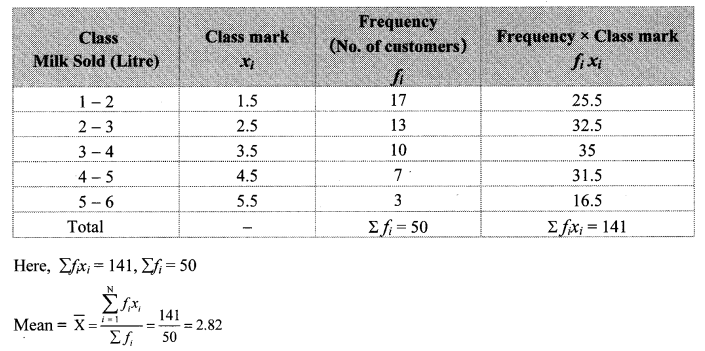∴ The mean of the milk sold is 2.82 litres.

Question 4.
A frequency distribution table for the production of oranges of some farm owners is given below. Find the mean production of oranges by ‘assumed mean’ method.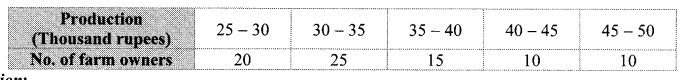Solution:
Let us take the assumed mean (A) = 37.5∴ The mean of the production of oranges is ₹ 35310.

Question 5.
A frequency distribution of funds collected by 120 workers in a company for the drought affected people are given in the following table. Find the mean of he funds by ‘step deviation’ method.Solution:
Here, we take A = 1250 and g = 500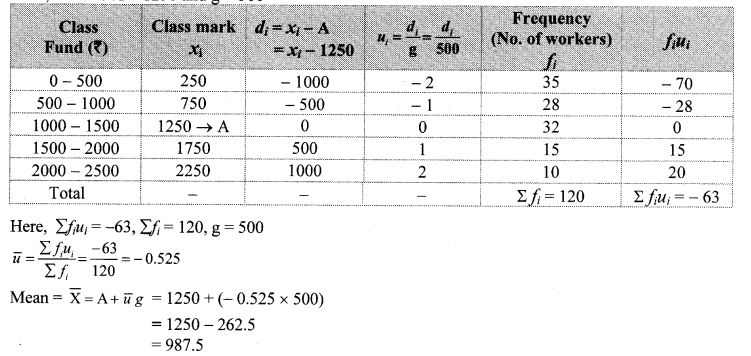∴ The mean of the funds collected is ₹ 987.5.

Question 6.
The following table gives the information of frequency distribution of weekly wages of 150 workers of a company. Find the mean of the weekly wages by ‘step deviation’ method.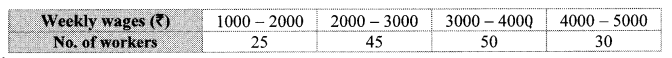Solution:
Here, we take A = 2500 and g = 1000.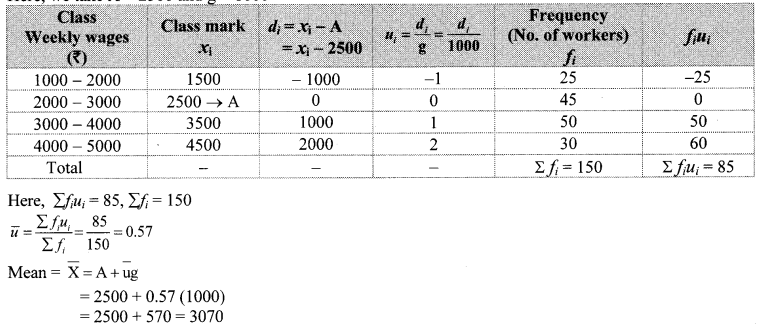∴ The mean of the weekly wages is ₹ 3070.

Question 1.
The daily sale of 100 vegetable vendors is given in the following table. Find the mean of the sale by direct method. (Textbook pg. no. 133 and 134)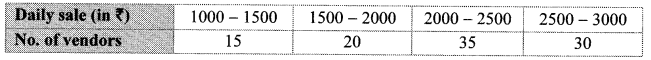Solution: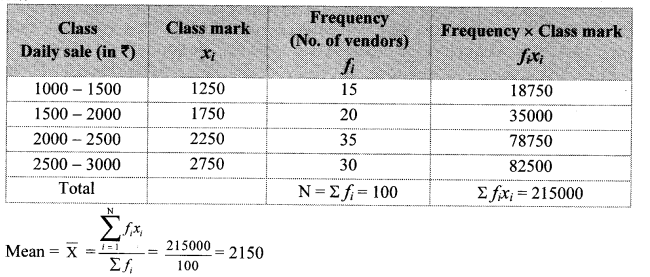The mean of the sale is 2150.

Question 2.
The amount invested in health insurance by 100 families is given in the following frequency table. Find the mean of investments using direct method and assumed mean method. Check whether the mean found by the two methods is the same as calculated by step deviation method (Ans: ₹ 2140). (Textbook pg. no. 135 and 136)Solution: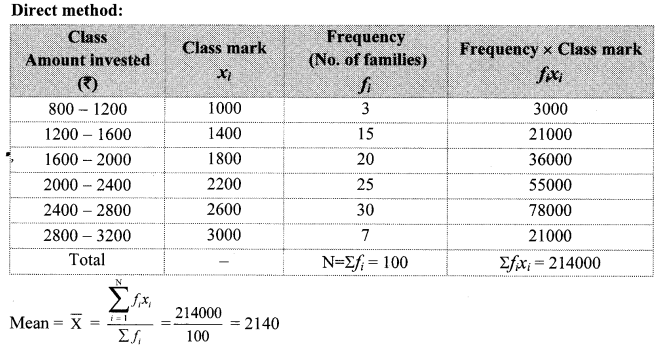∴ The mean of investments in health insurance is ₹ 2140.
Assumed mean method: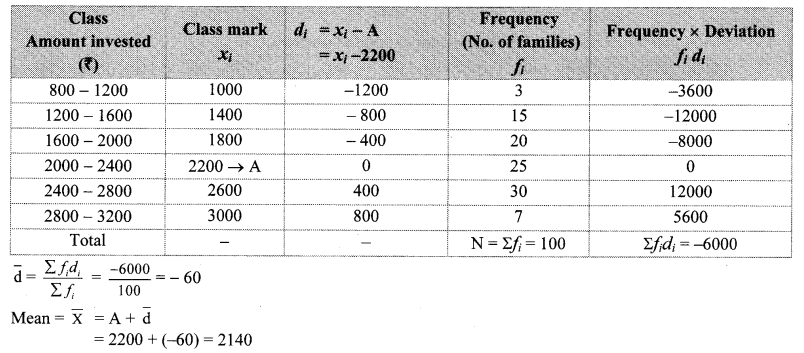∴ The mean of investments in health insurance is ₹ 2140.
∴ Mean found by direct method and assumed mean method is the same as calculated by step deviation method.

Question 3.
The following table shows the funds collected by 50 students for flood affected people. Find the mean of the funds.If the number of scores in two consecutive classes is very low, it is convenient to club them. So, in the above example, we club the classes 0 – 500, 500 – 1000 and 2000 – 2500, 2500 – 3000. Now the new table is as followsi. Solve by direct method.
ii. Verily that the mean calculated by assumed mean method is the same.
iii. Find the mean in the above example by taking A = 1750. (Textbook pg. no. 137)
Solution:
i. Direct method: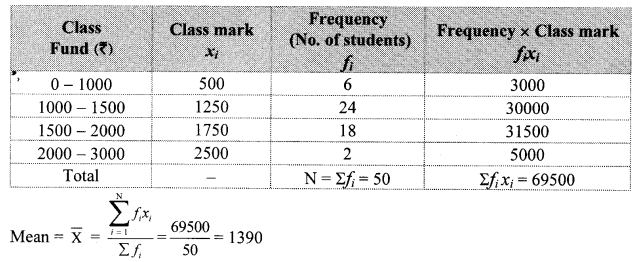∴ The mean of the funds is ₹ 1390.

ii. Assumed mean method:
Here, A = 1250∴ The mean calculated by assumed mean method is the same.

iii. Step deviation method:
Here, we take A = 1750 and g = 250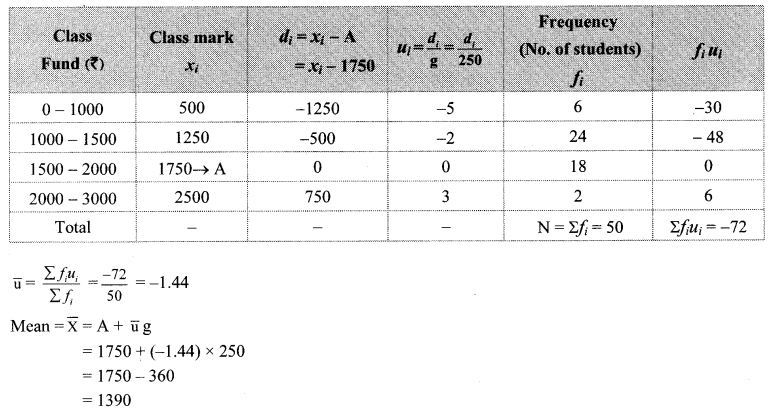∴ The mean of the funds is ₹ 1390.